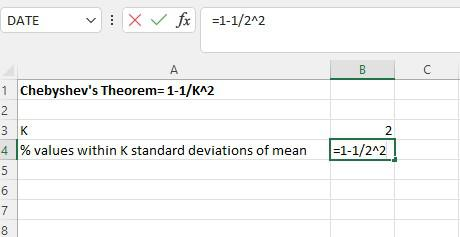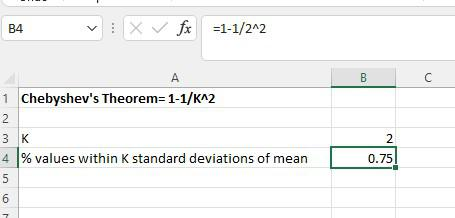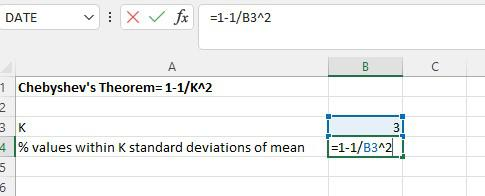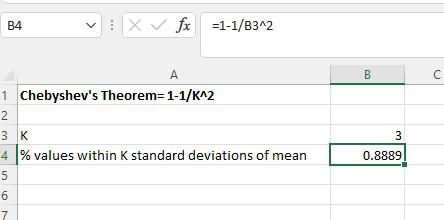Open in App
Not now

# How to Apply Chebyshev’s Theorem in Excel?

• Last Updated : 22 Aug, 2022

Chebyshev’s Theorem expresses that for any number k more noteworthy than 1, something like 1 – 1/k2 of the information values in any molded conveyance exist in k standard deviations of the mean. Chebyshev’s Theorem assists you with figuring out where the vast majority of your information falls inside a circulation of values. This hypothesis gives accommodating outcomes when you have just the mean and standard deviation. You don’t have to know the circulation of your information follows. For instance, for any molded appropriation, something like 1 – 1/42 = 93.75% of the qualities in the conveyance will exist in 4 standard deviations of the mean. This instructional exercise outlines a few instances of how to apply Chebyshev’s Theorem in Excel.

## Applying Chebyshev’s Theorem in Excel

Example 1: Use Chebyshev’s Theorem to find what percentage of values will fall between 20 and 60 for a dataset with a mean of 40 and a standard deviation of 10. To begin with, decide the incentive for k. We can do this by figuring out the number of standard deviations away 20 and 60 that are from the mean:

(20 – mean) / standard deviation = (20 – 40) / 10 = -20 / 10 = -2

(60 – mean) / standard deviation = (60 – 40) / 10 = 20 / 10 = 2

The qualities 20 and 60 are 2 standard deviations underneath or more than the mean, individually. In this way, k = 2. We can then utilize the accompanying equation in Excel to find the base level of values that fall inside 2 standard deviations of the mean for this dataset:Will get the result as below:The level of values that fall between 20 and 60 for this dataset will be something like 75%.

Example 2: Use Chebyshev’s Theorem to find what percentage of values will fall between 10 and 40 for a dataset with a mean of 25 and a standard deviation of 5. To begin with, decide the incentive for k. We can do this by figuring out the number of standard deviations away 10 and 40 that are from the mean:

(10 – mean) / standard deviation = (10 – 25) / 5 = -15 / 5 = -3

(40 – mean) / standard deviation = (40 – 25) / 5 = 15 / 5 = 3

The qualities 10 and 40 are 3 standard deviations underneath or more than the mean, individually. Hence, k = 3. We can then utilize the accompanying recipe in Excel to find the base level of values that fall inside 3 standard deviations of the mean for this dataset:The result will get as shown below:The level of values that fall between 10 and 40 for this dataset will be something like 88.89%.

My Personal Notes arrow_drop_up
Related Articles